U.S. Department of Transportation
1200 New Jersey Avenue, SE
Washington, DC 20590
202-366-4000

Federal Highway Administration Research and Technology
Coordinating, Developing, and Delivering Highway Transportation Innovations

 REPORT This report is an archived publication and may contain dated technical, contact, and link information
 Federal Highway Administration > Publications > Research Publications > 15065 > Methodology
 Publication Number:  FHWA-HRT-15-065    Date:  September 2015
 Publication Number: FHWA-HRT-15-065 Date: September 2015

# Safety Evaluation of Wet-Reflective Pavement Markings

## Chapter 3. Methodology

The EB methodology for observational before–after studies was used for the evaluation. This methodology is considered rigorous in that it accounts for regression-to-the-mean using a reference group of similar but untreated sites. In the process, safety performance functions (SPFs) were used to address the following issues:

• Overcoming the difficulties of using crash rates in normalizing for volume differences between the before and after periods.

• Accounting for time trends.

• Reducing the level of uncertainty in the estimates of safety effect.

• Properly accounting for differences in crash experience and reporting practice in amalgamating data and results from diverse jurisdictions.

The methodology also provided a foundation for developing guidelines for estimating the likely safety consequences of a contemplated strategy. The SPFs for roadways without wet-reflective markings were used with observed crash histories to estimate the number of crashes without treatment, and the CMFs developed were applied to this number to estimate the number without treatment.

In the EB approach, the estimated change in safety for a given crash type at a site is given by the equation in figure 1.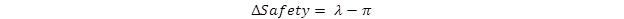Figure 1. Equation. Estimated change in safety.

Where:

λ = Expected number of crashes that would have occurred in the after period without the strategy.
π = Number of reported crashes in the after period.

In estimating λ, the effects of regression-to-the-mean and changes in traffic volume were explicitly accounted for using SPFs, which relate crashes of different types to traffic flow and other relevant factors for each jurisdiction based on untreated sites (reference sites). Annual SPF multipliers were calibrated to account for temporal effects on safety (e.g., variation in weather, demography, and crash reporting).

In the EB procedure, the SPF was used to first estimate the number of crashes that would be expected in each year of the before period at locations with traffic volumes and other characteristics similar to the one being analyzed (i.e., reference sites). The sum of these annual SPF estimates (P) was then combined with the count of crashes (x) in the before period at a strategy site to obtain an estimate of the expected number of crashes (m) before the strategy was applied. This estimate of m was calculated using the equation in figure 2.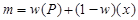Figure 2. Equation. Empirical Bayes estimate of expected crashes.

Where the EB weight, w, was estimated from the mean and variance of the SPF estimate using the equation in figure 3.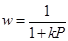Figure 3. Equation. Empirical Bayes weight.

Where:

k = Constant for a given model and is estimated from the SPF calibration.

In the SPF calibration process, a negative binomial distributed error structure was assumed with k being the overdispersion parameter of this distribution.

A factor was then applied to m to account for the length of the after period and differences in traffic volumes between the before and after periods. This factor was the sum of the annual SPF predictions for the after period divided by P, the sum of these predictions for the before period. The result, after applying this factor, was an estimate of λ. The procedure also produced an estimate of the variance of λ.

The estimate of λ was then summed over all sites in a strategy group of interest (to obtain λsum ) and compared with the count of crashes observed during the after period in that group ( πsum ). The variance of λ was also summed over all sites in the strategy group.

The index of effectiveness (θ) was estimated using the equation in figure 4.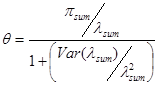Figure 4. Equation. Index of effectiveness.

The standard deviation of θ was given by the equation in figure 5.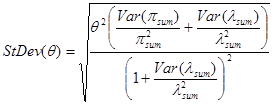Figure 5. Equation. Standard deviation of index of effectiveness.

The percent change in crashes is calculated as 100(1 - θ ); thus a value of θ = 0.7 with a standard deviation of 0.12 indicates a 30-percent reduction in crashes with a standard deviation of 12 percent.